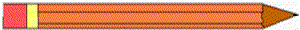Skill 25E Centimeters: Estimate and Measure Without using a ruler - answer the following: About how many centimeters long is the pencil?about 3 centimeters long about 8 centimeters about 15 centimeters Now use a centimeter ruler to measure the pencil.  About how many centimeters long is the pencil? about 3 centimeters long about 8 centimeters long about 15 centimeters long